# Thread: differentiation question

1. ## differentiation question

Hello, how would i go about solving this equation?

z = (6x^4 - 6x^-3 - 2x + 5)^-2

im a little confused with the ^-2 at the end

im only supposed to find it to the first derivative

Also how would i go about solving this one to the first derivative?

x^2 e^3x^2-4x^3-3x+8 (its all one power, e *to the power of 3x * then 3x to the power of 2 .......)

i have this

dy/dx = 2x e^6x - 12x^2 - 3

2. Hello, how would i go about solving this equation?

$\displaystyle z = (6x^{4} - 6x^{-3} - 2x + 5)^{-2}$

im a little confused with the ^-2 at the end

im only supposed to find it to the first derivative
Use the chain rule.

$\displaystyle -2(6x^{4}-6x^{-3}-2x+5)^{-3}(24x^{3}+18x^{-4}-2)$

Now, simplify.

3.Originally Posted by entrepreneurforum.co.ukHello, how would i go about solving this equation?

z = (6x^4 - 6x^-3 - 2x + 5)^-2

im a little confused with the ^-2 at the end

im only supposed to find it to the first derivative
First, get the terminology right. You're not "solving an equation", you're differentiating a function. So the question should be "How would I go about differentiating this function?"

Use the chain rule. The function z is of the form $\displaystyle z=y^{-2}$, where $\displaystyle y = 6x^4 - 6x^-3 - 2x + 5$. The derivative of $\displaystyle y^{-2}$ is $\displaystyle -2y^{-3}$, and the chain rule says that you must multiply this by the derivative of y.Originally Posted by entrepreneurforum.co.ukAlso how would i go about solving this one to the first derivative?

x^2 e^3x^2-4x^3-3x+8 (its all one power, e *to the power of 3x * then 3x to the power of 2 .......)

i have this

dy/dx = 2x e^6x - 12x^2 - 3
You got the $\displaystyle -4x^3-3x+8$ part right, so let's concentrate on differentiating the $\displaystyle x^2 e^{3x^2}$ bit. That is a product of two components, $\displaystyle x^2$ times $\displaystyle e^{3x^2}$. To differentiate it, you must use the product rule. That is not what you have done. Look up what the product rule for differentiation says, and have another go at this.

4. And, just in case a picture helps...

The chain rule generally...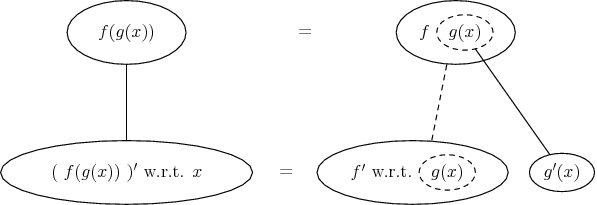... in which straight continuous lines differentiate downwards with respect to x, and the straight dashed line with respect to the dashed balloon expression. In this case...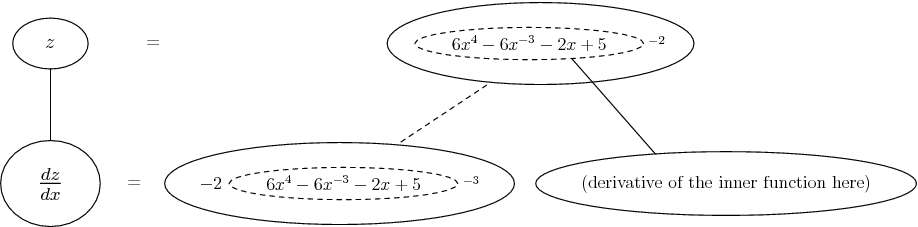Introducing also the product rule thus...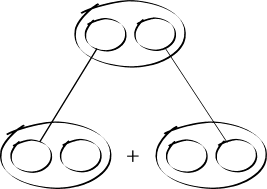or thus...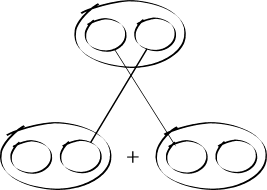and in this case, incorporating the chain rule, too...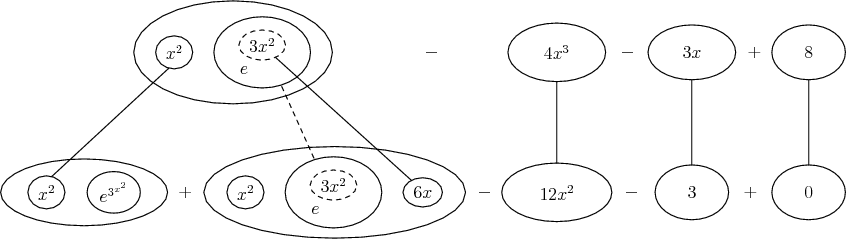Don't integrate - balloontegrate! Balloon Calculus: worked examples from past papers

5. Opalg
this is what i have now for the second question

(x^2) d(e^3x^2) + d(x^2) (e^3x^2)

= (3^2)(6e^5x) + (2x) (e^3x^2)

is this right?

6.Originally Posted by entrepreneurforum.co.ukOpalg
this is what i have now for the second question

(x^2) d(e^3x^2) + d(x^2) (e^3x^2)

= (3^2)(6e^5x) + (2x) (e^3x^2)

is this right?
That's a step in the right direction. You are now applying the product rule correctly, but there's still a problem with differentiating e^3x^2.

Part of the problem is that you are interpreting the ambiguous expression e^3x^2 as though it means (e^3x)^2 = e^(6x). But I think it is much more likely to mean e^(3x^2). There is a difference between $\displaystyle (e^{3x})^2$ and $\displaystyle e^{3x^2}$, and you need to make sure that you are using the right one.

In either case, you need to use the chain rule to differentiate $\displaystyle e^y$ (where y is either 6x or 3x^2). The answer that you have given above, 6e^(5x), is wrong in either case.

7. galactus

is this right?

-2(6x^4 - 6x^-3 - 2x +5) ^-3 (24x^3 +18x^-4 -2)

-2(24x + 18x - 2x + 3)^-3

do the power signs cancel each other out?

also im a little confused on this question, when finding the stationary points

y = (3- x)^3

I'm able to do these type of questions, but its the same problem as im getting with the question i asked earlier, about the power signs, so far i've got to

3(3-x)^2 (-x) = 0

and im just getting confused on where to go from there, any help would be great.

8. opalg it does mean (e)^(3x^2)

would it be something like...

3x^2 (e) ^(3x^2 -1)

im getting a little lost with this area of differentiation now, is this going in the right direction?

9. also thanks to everyone whos helped so far, im getting it alot more than what i did get before this thread, thanks

10.Originally Posted by entrepreneurforum.co.ukit does mean (e)^(3x^2)

would it be something like...

3x^2 (e) ^(3x^2 -1)

im getting a little lost with this area of differentiation now, is this going in the right direction?
No, still not there.The derivative of $\displaystyle e^x$ is $\displaystyle e^x$, not $\displaystyle e^{x-1}$. And the derivative of 3x^2 is 6x. So by the chain rule the derivative of $\displaystyle e^{3x^2}$ is $\displaystyle 6xe^{3x^2}$.

11. ok im trying to do this problem, and im still getting lost

$\displaystyle 3x^2 e$^$\displaystyle (3x^2 -5x^2 - 3x + 6 - 52)$

where everything after e^ in brackets is to the power of e

12.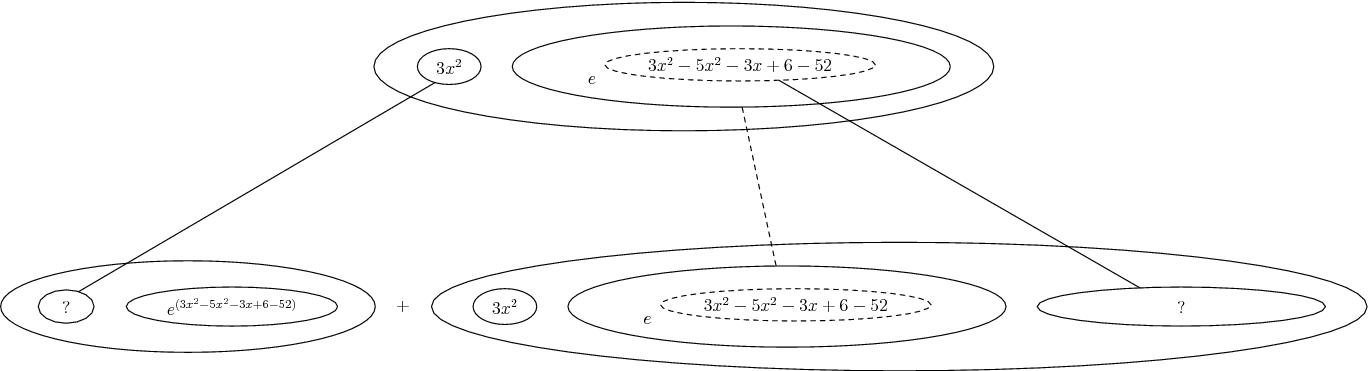Don't integrate - balloontegrate! Balloon Calculus: worked examples from past papers

13. tom im not sure what your trying to tell me, i kind of have an idea, but i know how to differentiate equations, just really stuck when it comes to the e^x function, as far as i know you don't differentiate the exponential function? so what i have is

$\displaystyle 6x$ $\displaystyle e$^$\displaystyle 3x^2-5x^2 - 3x + 6 -52$

and what i think you tom are trying to show me is the product rule, and im not sure it applies here? i might be wrong?

14.Originally Posted by entrepreneurforum.co.uk
...as far as i know you don't differentiate the exponential function?
Well, you do - what you've noticed is that the derivative is of $\displaystyle e^x$ is $\displaystyle e^x$. However, differentiating e to the power of anything more
complicated than x is going to need the chain rule. So you'll have as your derivative e to that same (complicated) power but the
whole thing then multiplied by the derivative of that (complicated) power.Originally Posted by entrepreneurforum.co.uk
...and what i think you tom are trying to show me is the product rule, and im not sure it applies here?
It always does apply if you're differentiating a product, as here - i.e. the product of $\displaystyle 3x^2$ and e to that horrible power.
Zooming out of the chain rule to show the product rule alone...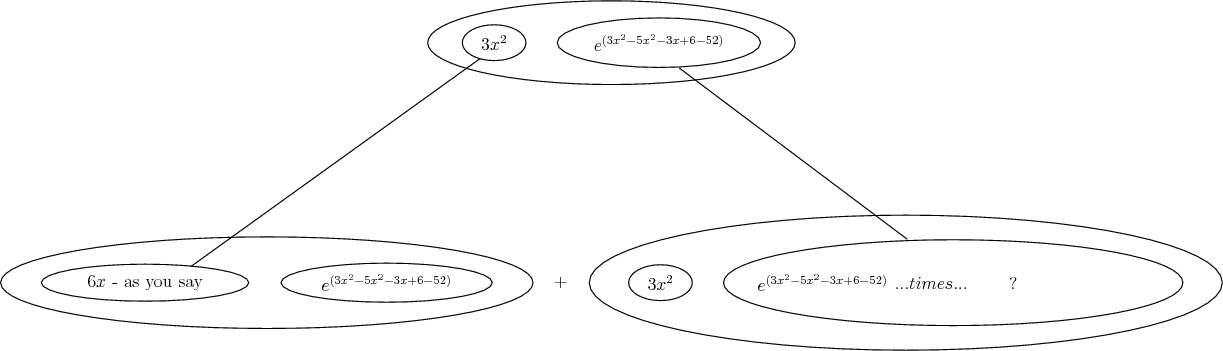Don't integrate - balloontegrate! Balloon Calculus: worked examples from past papers

15. thanks tom im slowly getting it more and more!

what i have now is

(3x^2(e^6x-10x-3) + (6x)(e^3x^2-5x^2-3x+6-52)

right or wrong?

#### Search Tags

differentiation, question